# Look at the normal curve below, and find \mu,\ \mu+\sigma, and \sigmaZSlilyta79jd 2021-11-18 Answered
Look at the normal curve below, and find $$\displaystyle\mu,\ \mu+\sigma$$, and $$\displaystyle\sigma$$
$\begin{array}{|c|c|}\hline \mu & = & \\ \hline \mu+\sigma & = & \\ \hline \sigma & = & \\ \hline \end{array}$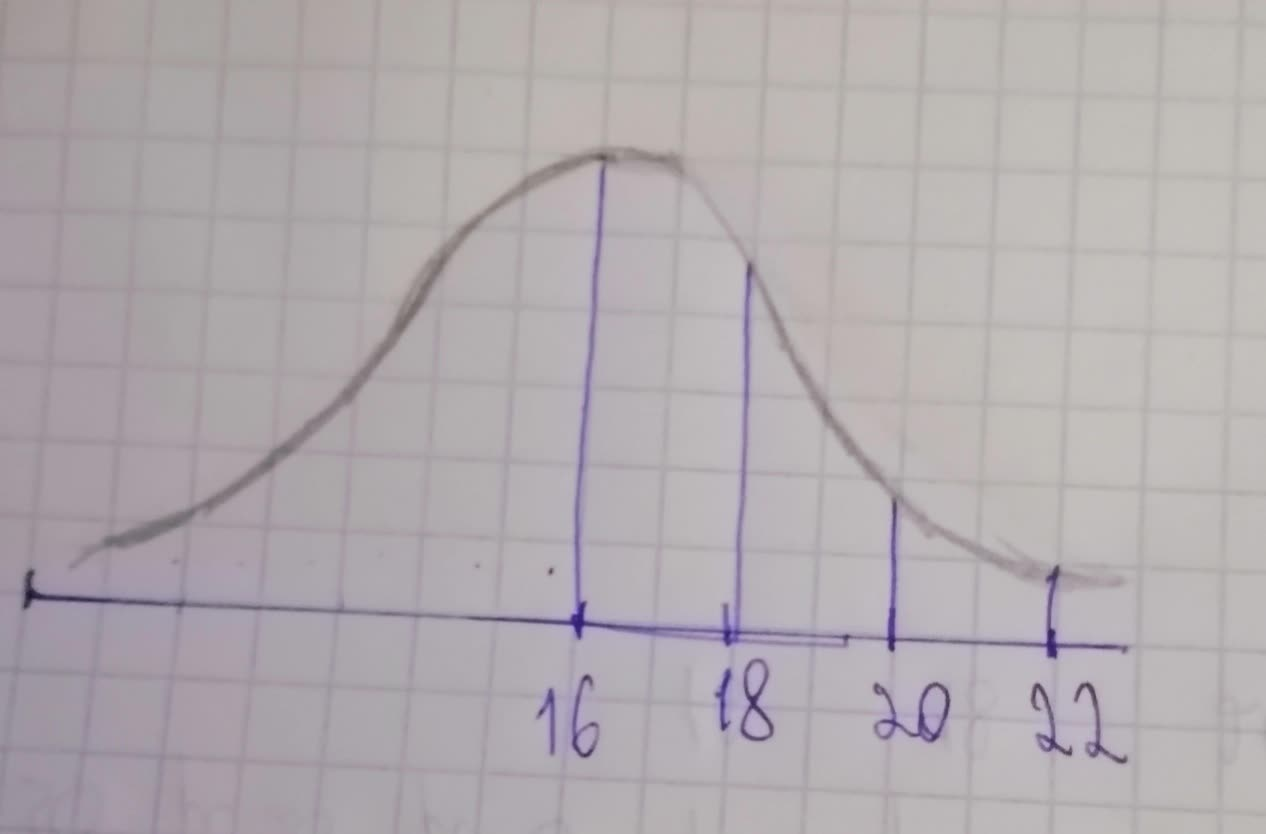• Questions are typically answered in as fast as 30 minutes

### Plainmath recommends

• Get a detailed answer even on the hardest topics.
• Ask an expert for a step-by-step guidance to learn to do it yourself.SaurbHurbkj

Step 1
The value of mean $$\displaystyle{\left(\mu\right)}$$ is obtained below:
The below graph represents the normal curve with the one standard deviation, two standard deviations and three standard deviations either side of the mean with their respective values.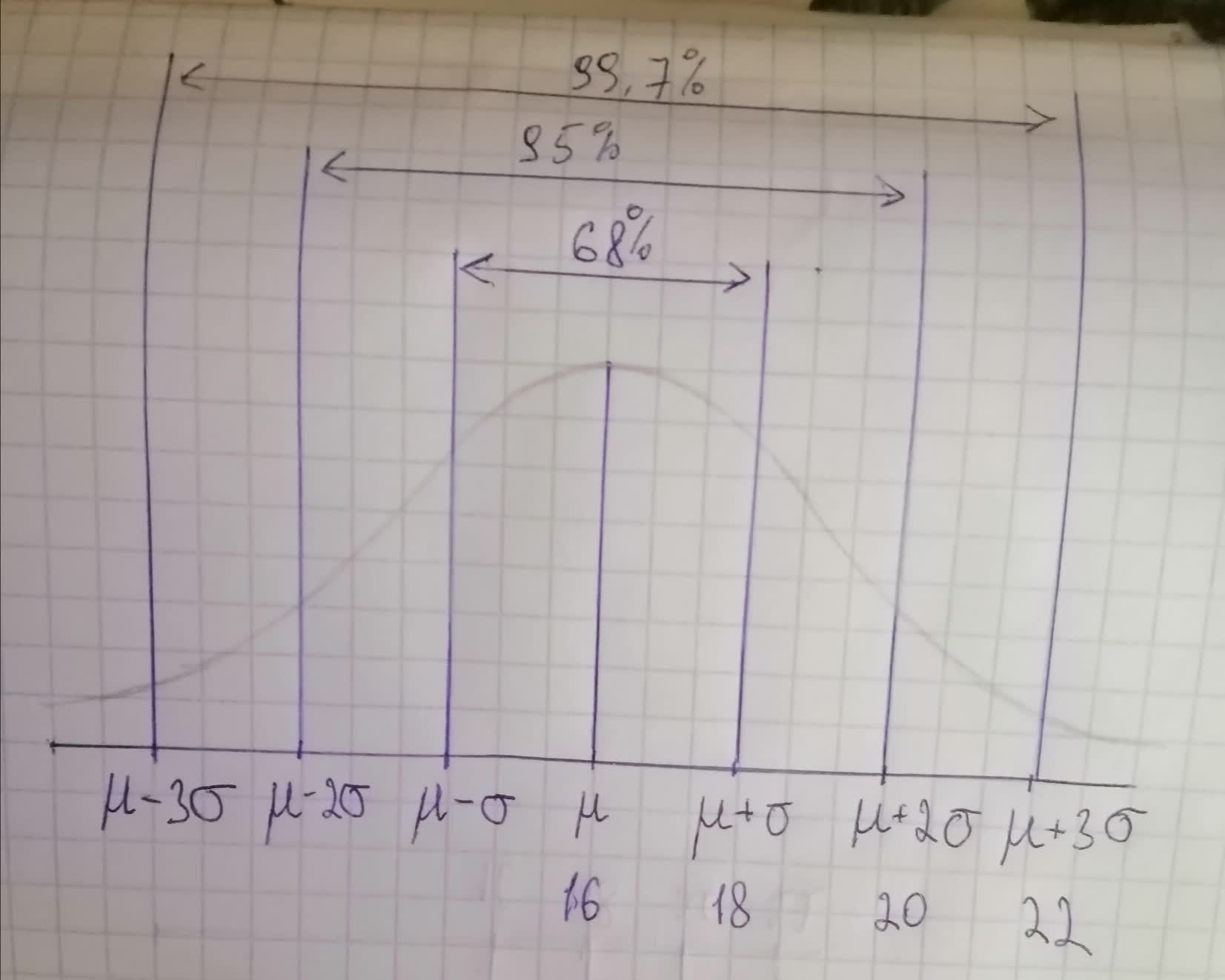From the graph it is clear that, parameter that lies in the center of the normal curve can be identified as the population mean $$\displaystyle{\left(\mu\right)}$$ which is 16.
The value of population mean $$\displaystyle{\left(\mu\right)}$$ is 16.
The value of mean $$\displaystyle{\left(\mu\right)}$$ is obtained by using the concept of the empirical rule.
Step 2
The value of one standard deviation away from the mean $$\displaystyle{\left(\mu+\sigma\right)}$$ is obtained below:
The below graph represents the normal curve with the one standard deviation, two standard deviations and three standard deviations either side of the mean with their respective values.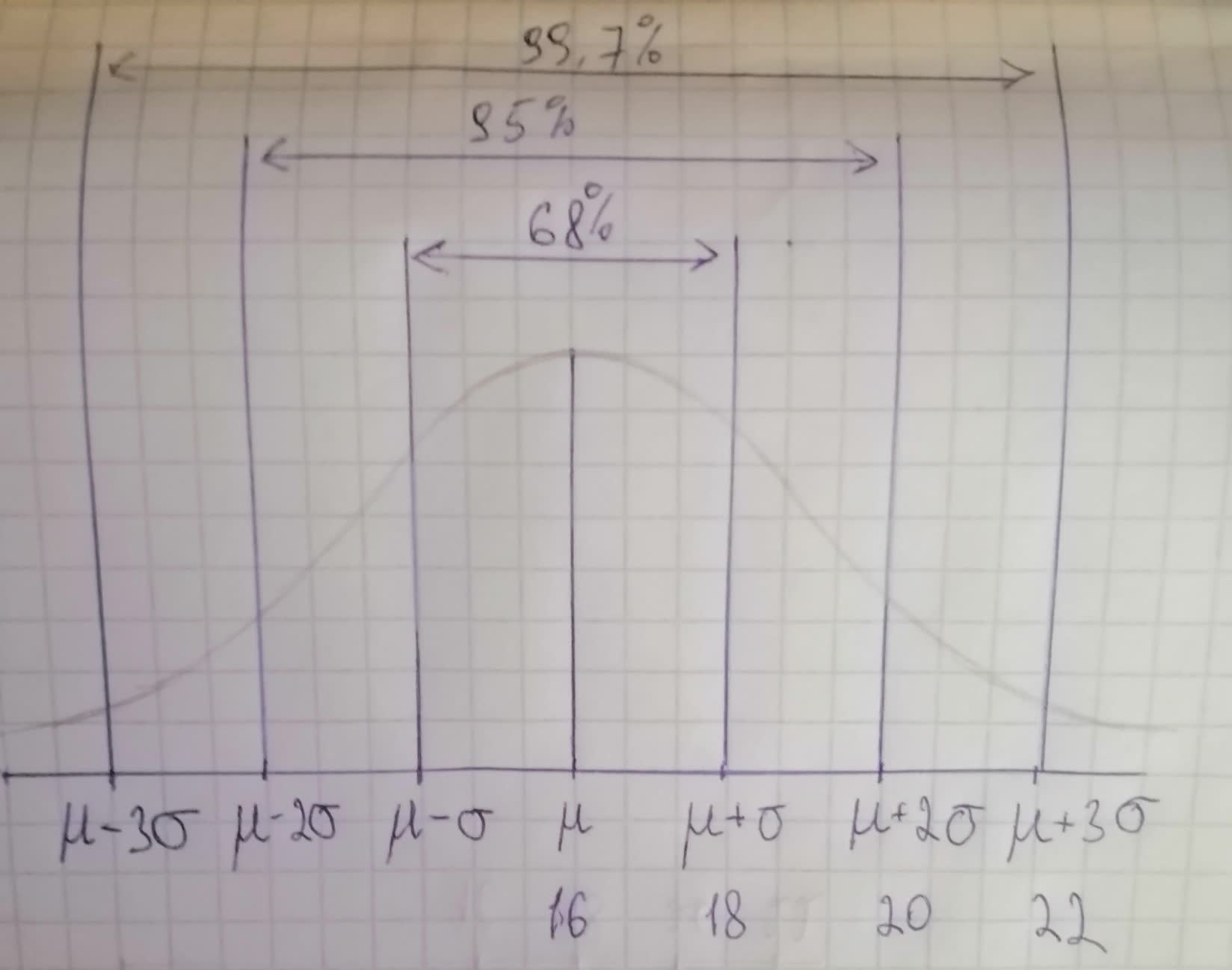By the empirical rule, one standard deviation away from the mean can be identified as $$\displaystyle{\left(\mu+\sigma\right)}$$ which is 18.
The value of one standard deviation away from the mean is, $$\displaystyle\mu+\sigma={18}$$
The value of one standard deviation away from the mean PSK(\mu+\sigma) is obtained by using the concept of the empirical rule.
Step 3
The value of population standard deviation is obtained below:
The below graph represents the normal curve with the one standard deviation, two standard deviations and three standard deviations either side of the mean with their respective values.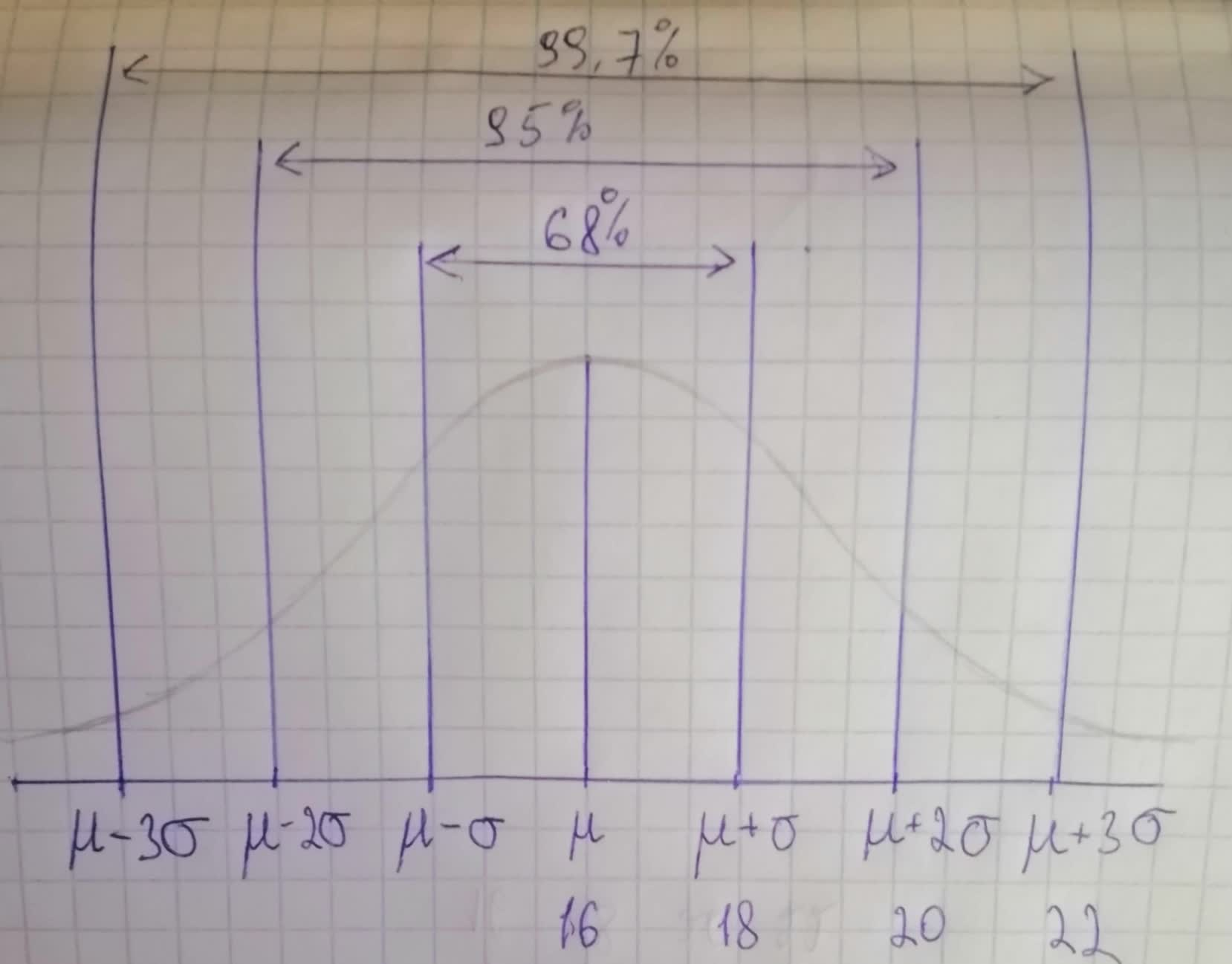It is clear that, the value of one standard deviation away from the mean $$\displaystyle{\left(\mu+\sigma\right)}$$
is 18 and the value of the population mean $$\displaystyle{\left(\mu\right)}$$ is 16.
Consider:
$$\displaystyle\mu+\sigma={18}$$
$$\displaystyle{16}+\sigma={18}$$
$$\displaystyle\sigma={18}-{16}$$
$$\displaystyle\sigma={2}$$
The value of population standard deviation $$\displaystyle{\left(\sigma\right)}$$ is 2.
The value of population standard deviation $$\displaystyle{\left(\sigma\right)}$$ is obtained by the difference between the values of one standard deviation away from the mean $$\displaystyle{\left(\mu+\sigma\right)}$$ to the population mean.
###### Have a similar question?Margaret Plemons

Step 1
Look at the normal curve below, and find $$\displaystyle\mu,\ \mu+\sigma$$, and $$\displaystyle\sigma$$.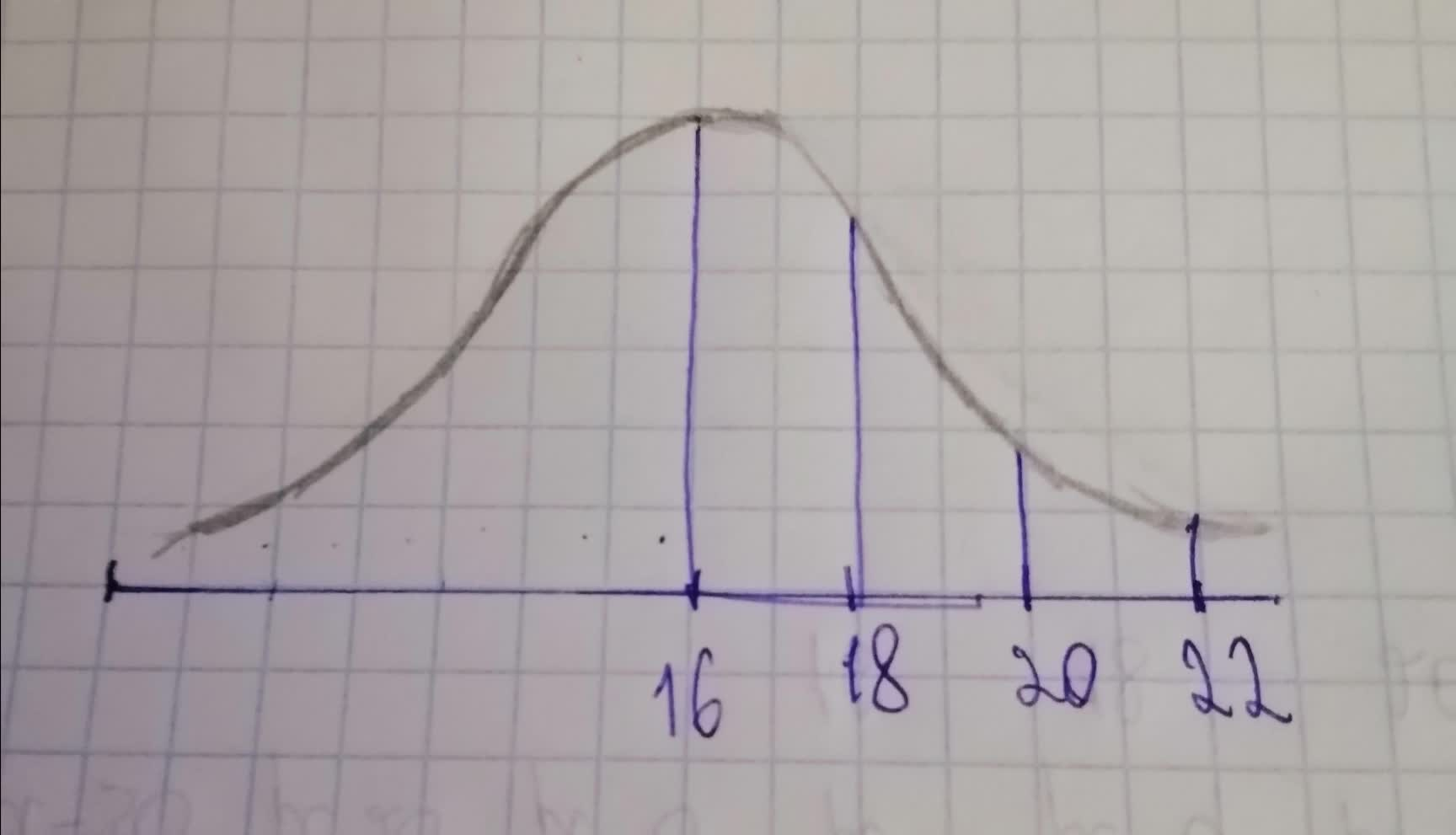from above curve:
$$\displaystyle\mu=$$ central value on the curve =16
$$\displaystyle\mu+\sigma=$$ 1 standard deviaiton greater value from mean =18
$$\displaystyle\sigma={18}-{16}={2}$$